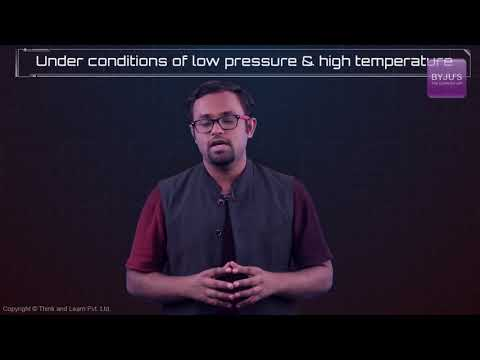# Derivation of Ideal Gas Equation

## What is Ideal Gas?

An ideal gas is a theoretical gas composed of a set of randomly-moving point particles that interact only through elastic collisions.

The ideal gas concept is useful because it obeys the ideal gas law, a simplified equation of state and is amenable to analysis under statistical mechanics.

## Recommended Videos## Ideal Gas Laws

The laws which deal with ideal gases are naturally called ideal gas laws and the laws are determined by the observational work of Boyle in the seventeenth century and Charles in the eighteenth century.

• Boyles Law – states that for a given mass of gas held at a constant temperature the gas pressure is inversely proportional to the gas volume.
• Charles Law – states that for a given fixed mass of gas held at a constant pressure the gas volume is directly proportional to the gas temperature.

## Ideal Gas Equation

The Ideal gas law is the equation of state of a hypothetical ideal gas. It is a good approximation to the behaviour of many gases under many conditions, although it has several limitations. The ideal gas equation can be written as

PV = nRT

Where,

• P is the pressure of the ideal gas.
• V is the volume of the ideal gas.
• n is the amount of ideal gas measured in terms of moles.
• R is the universal gas constant.
• T is the temperature.

According to the Ideal Gas equation-

The product of Pressure & Volume of a gas bears a constant relation with the product of Universal gas constant and the temperature.

i.e. $pv = nRT$

## Universal Gas Constant (R)

When the molecular mass of any gas is multiplied by its specific gas constant (R), it is observed that the product R is always the same for all gases. This product is called universal gas constant and it is denoted as R.

Note: From the SI system the value of the universal gas constant is 8.314 kJ/mole.K

## Derivation of the Ideal Gas Equation

Let us consider the pressure exerted by the gas to be ‘p,

The volume of the gas be – ‘v

Temperature be – T

n – be the number of moles of gas

Universal gas constant – R

According to Boyle’s Law,

At constant n & T, the volume bears an inverse relation with the pressure exerted by a gas.

i.e. $v \propto \frac{1}{p}$ ………………………………(i)

According to Charles’ Law,

When p & n are constant, the volume of a gas bears a direct relation with the Temperature.

i.e. $v \propto T$ ………………………………(ii)

When p & T are constant, then the volume of a gas bears a direct relation with the number of moles of gas.

i.e. $v \propto n$ ………………………………(iii)

Combining all the three equations, we have-

$v \propto \frac{nT}{p}$

or $pv = nRT$

where R is the Universal gas constant, which has a value of 8.314 J/mol-K

1. Nice